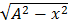# A particle executes simple harmonic motion with an amplitude of 5 cm. When the particle is at 4 cm from the mean position, the magnitude of its velocity in SI units is equal to that of its acceleration. Then, its periodic time in seconds is: (a)(b)(c)(d)## Question ID - 50265 :- A particle executes simple harmonic motion with an amplitude of 5 cm. When the particle is at 4 cm from the mean position, the magnitude of its velocity in SI units is equal to that of its acceleration. Then, its periodic time in seconds is: (a)(b)(c)(d)3537

V=…(1)

A=−2x                                       … (2)

|v|=|a|                                        … (3)=x

A2−x2=x2

52−42=(42)

⇒ 3=×4

T=2/Next Question :
 Consider a Young’s double slit experiment as shown in figure. What should be the slit separation d in terms of wavelengthsuch that the first minima occurs directly in front of the slit (S1)?(a)(b)(c)(d)